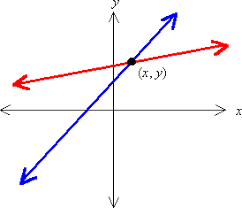# Solving a System of Equations by Substitution and Elimination

A system of equations involves trying to solve for more than one variable. What this means is that a system of equations helps you to see how to different equations relate or where they intersect if you were to graph them.

There are several different ways to solve a system of equations. In this post, we will solve y using the substitution and the elimination methods.Substitution

Substitution involves choosing one of the two equations and solving for one variable. Once this is done we substitute the expression into the equation for which we did not solve a variable for. When this is done the second equation only has one unknown variable and this is basic algebra to solve.

The explanation above is abstract so here is a mathematical example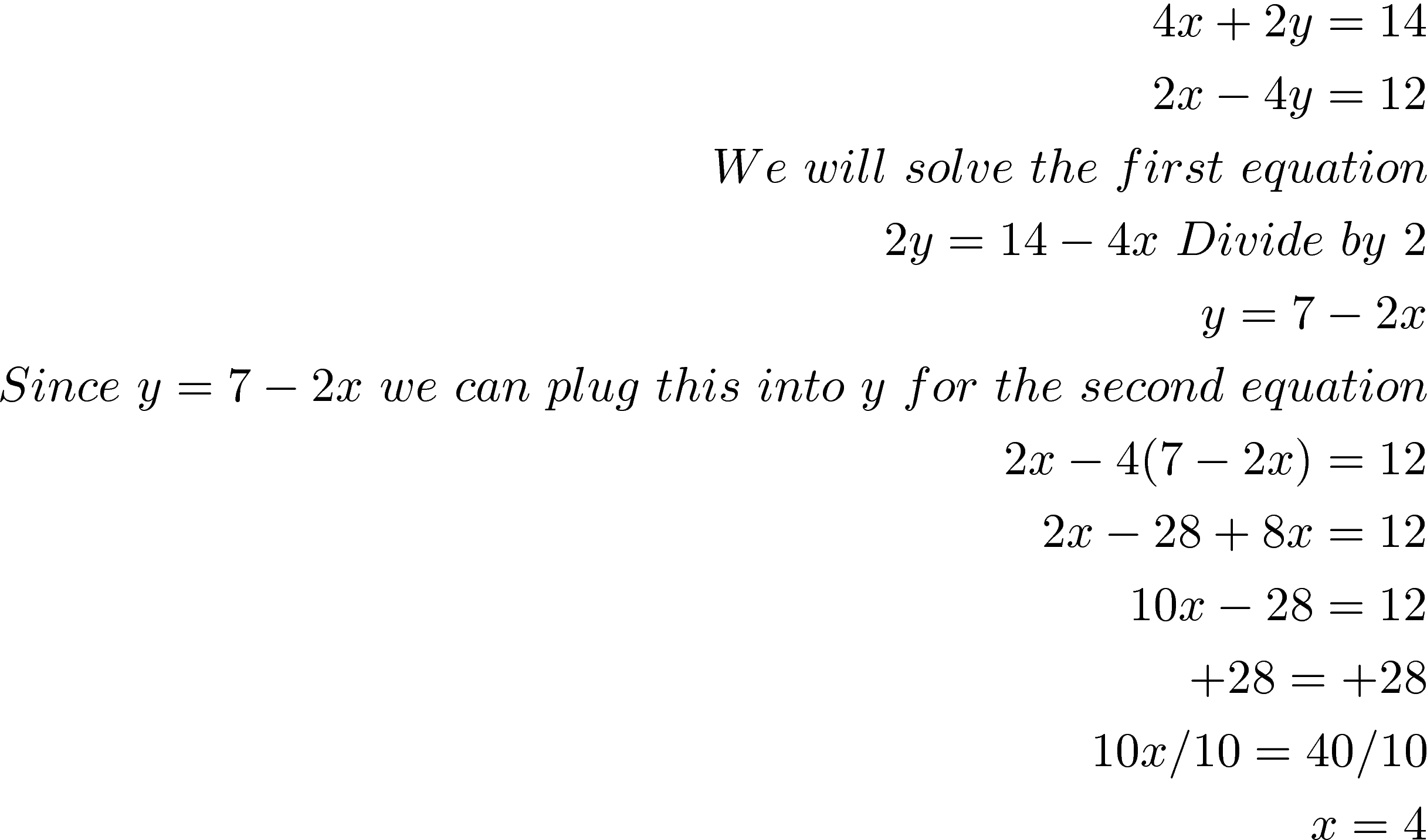We are not done. We now need to use are x value to find our y value. We will use the first equation and replace x to find y.This means that our ordered pair is (4, -1) and this is the solution to the system. You can check this answer by plugging both numbers into the x and y variable in both equations.

Elimination

Elimination begins with two equations and two variables but eliminates one variable to have one equation with one variable. This is done through the use of the addition property of equality which states when you add the same quantity to both sides of an equation you still have equality. For example 2+2 = 2 and if at 5 to both sides I get 7 + 7 = 7. The equality remains.

Therefore, we can change one equation using the addition property of equality until one of the variables has the same absolute value for both equations. Then we add across to eliminate one of the variables. If one variable is positive in one equation and negative in the other and has the same absolute value they will eliminate each other. Below is an example using the same system of equations as the previous example.

.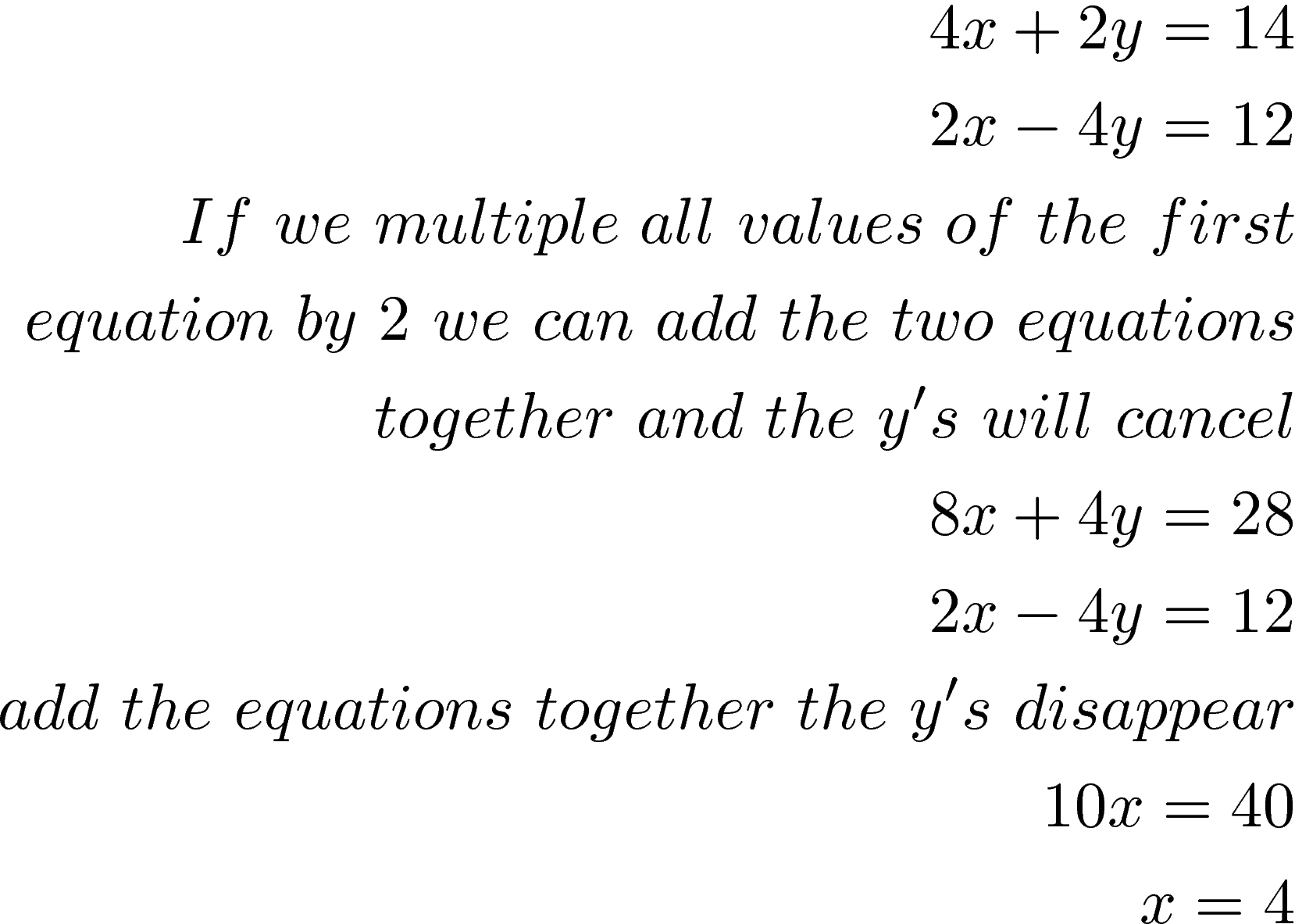You can take the x value and plug it into y. We already know y =1 from the previous example so we will skip this.

There are also times when you need to multiply both equations by a constant so that you can eliminate one of the variables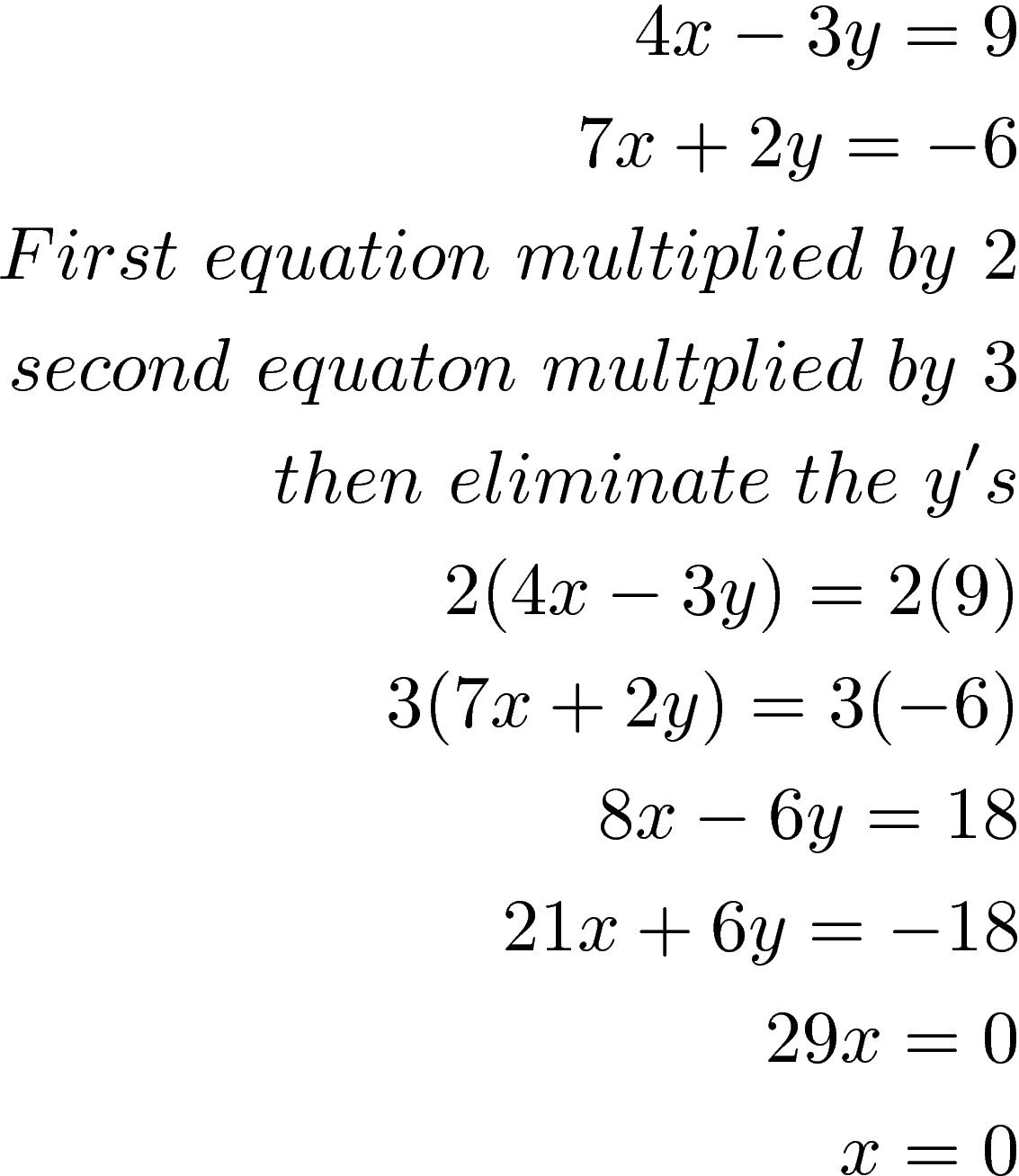We now replace x with 0 in the second equation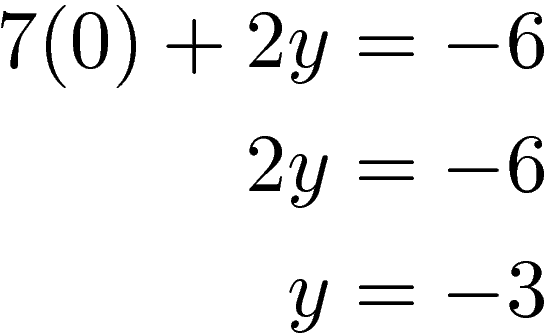Our ordered pair is (0, -3) which also means this is where the two lines intersect if they were graphed.

Conclusion

Solving a system of equations allows you to handle two variables (or more) simultaneously. In terms of what method to use it really boils down to personal choice as all methods should work. Generally, the best method is the one with the least amount of calculation.